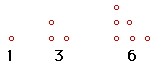# Triangular Numbers

Consider the pattern formed by these dots.The number used to describe each “triangle” is called a triangular number. Given a number, can you arrange that many dots into a triangle? If you can, you have identified a triangular number. The sequence of triangular numbers starts with 1, 3, 6, and so on.

Draw a picture of the next triangle in the sequence and state the next triangular number.

Without drawing any more triangles, determine the next five triangular numbers and explain how you found your results.Extension
What is the 100th triangular number? Explain your result.